Processing ......FreeComputerBooks.com Links to Free Computer, Mathematics, Technical Books all over the World

Elementry, High School, Technical, and Engineering Mathematics
Related Book Categories:
•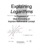Explaining Logarithms (Dan Umbarger)

This book shows the evolution of logarithmic ideas over 350 years. The author do believes that a quick review of mathematics as it was practiced for hundreds of years would be helpful for many students in understanding logarithms as they are still used today.

•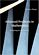Advanced Problems in Mathematics: Preparing for University

This book is intended to help candidates prepare for entrance examinations in mathematics and scientific subjects, including STEP (Sixth Term Examination Paper). is recommended as preparation for any undergraduate mathematics course.

•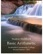Basic Arithmetic Student Workbook (Donna Gaudet, et al)

This workbook is designed to lead students through a basic understanding of numbers and arithmetic. It allows students to see the big picture of math and helps students recognize algebra as a natural extension of arithmetic.

•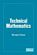Technical Mathematics (Morgan Chase)

This developmental-level mathematics textbook is intended for career-technical students. It provides a thorough review of pre calculus topics ranging from algebra, geometry, and trigonometry, with a strong emphasis on their applications in specific occupations.

•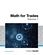Math for Trades: Volume 1 (Chad Flinn, et al.)

This volume represents the building blocks for math training. The goal of this volume is to get students prepared for the more advanced topics that they will encounter during their trades math education.

•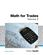Math for Trades: Volume 2 (Chad Flinn, et al.)

This volume continues where the Volume 1 left off. Volume 2 increases the challenge with topics such as converting units and working with equations, perimeter, area, and volume. Once again the material is presented from a trades perspective.

•Elementary Mathematics (William Chen, et al)

The overall emphasis of this book is on examples, of which there are many. The material has been organized in such a way to create a single volume suitable for preparing the student for university mathematics.

•High School Mathematics Extensions (Zhuo Jia Dai, et al)

This online textbook is aimed at, but not limited to, 14 to 18 year olds who are interested in mathematics in general. Several interesting topics not covered in the standard high school curriculum are introduced in this text.

•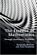The Essence of Mathematics Through Elementary Problems

This book consists of a sequence of 270 problems of increasing sophistication (Mental Skills; Arithmetic; Word Problems; Algebra; Geometry; Infinity) – with commentary and full solutions. The reader is assumed to have a reasonable grasp of school mathematics.

•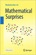Mathematical Surprises (Mordechai Ben-Ari)

This is open access book provides plenty of pleasant mathematical surprises. There are many fascinating results that do not appear in textbooks although they are accessible with a good knowledge of secondary-school mathematics.

•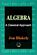Algebra: A Classical Approach (Jon Blakely)

This book provides a complete and contemporary perspective on classical polynomial algebra through the exploration of how it was developed and how it exists today, with discrete explanations from functions to quadratic and linear equations, etc.

•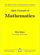Basic Concepts of Mathematics (Elias Zakon)

This book helps the student complete the transition from purely manipulative to rigorous mathematics, with topics that cover basic set theory, fields (with emphasis on the real numbers), a review of the geometry of three dimensions, and properties of linear spaces.

•Mathematics Concepts for Elementary School Teachers

This book helps pre-service teachers become more effective problem-solvers so they can better teach their students. It is appropriate for elementary school education programs for pre-service teachers.

•Mathematics for Elementary Teachers (Michelle Manes)

This book will help you to understand elementary mathematics more deeply, gain facility with creating and using mathematical notation, develop a habit of looking for reasons and creating mathematical explanations, etc.

•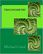Trigonometry (Michael Corral)

This is a text on elementary trigonometry. Though designed for college students, it could also be used in high schools. The traditional topics are covered, but a more geometrical approach is taken than usual.

•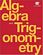Algebra and Trigonometry (Jay Abramson, et al)

This book provides a comprehensive and multi-layered exploration of algebraic principles. It is suitable for a typical introductory Algebra & Trigonometry course, and was developed to be used flexibly. The modular approach and the richness of content ensures that the book meets the needs of a variety of programs.

•Trigonometry (Ted Sundstrom, et al)

This college level trigonometry text may be different than most other trigonometry textbooks. Readers are expected to do more than read the book but is expected to study the material in the book by working out examples rather than just reading about them.

•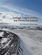College Trigonometry (Carl Stitz, et al)

This text provides a supportive environment to help students successfully learn the content of a standard trigonometry course. By incorporating interactive learning techniques, it helps students to better understand concepts, focus their studying habits, and obtain greater mathematical success.

•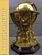Trigonometry (Richard Beveridge)

This book takes the torture out of trigonometry, explaining basic concepts in plain English and offering lots of easy-to-grasp example problems. It also explains the "why" of trigonometry, using real-world examples that illustrate the value of trigonometry.

•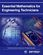Essential Mathematics for Engineering Technicians (OP-TEC)

This book explains mathematical jargon and develops the formulae used by engineers from first principles. The first chapter is a summary so the reader can quickly see where further study is needed. The book is in two parts, pure and applied.

•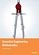Essential Engineering Mathematics (Michael Batty)

The aim is more to highlight and explain some areas commonly found difficult, such as calculus, and to ease the transition from school level to university level mathematics, where sometimes the subject matter is similar, but the emphasis is usually different.

•Elementary College Geometry (Henry Africk)

This text is intended for a brief introductory course in plane geometry, covers the topics from elementary geometry that are most likely to be required for more advanced mathematics courses. The only prerequisite is a semester of algebra.

•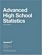Advanced High School Statistics (David M Diez, et al)

This book is geared to the high school audience and is specifically tailored to be aligned with the AP Statistics curriculum. It is already being used by many high schools and community colleges throughout the country.

•Mathematics Illuminated (MacGregor Campbell)

This book is a 13-part, integrated-media resource created for adult learners and high school teachers. The series covers the broad scope of human knowledge through the study of mathematics and its relevance in the world today.

•Six Septembers: Mathematics for the Humanist (Patrick Juola)

Developing the skills to enable humanist scholars to address complicated technical material with confidence. Mathematics operates under a regime of shared assumptions, and it is our purpose to elucidate some of those assumptions for the newcomer.

•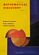Mathematical Discovery (A.M. Bruckner, et al)

A course introducing the idea of mathematical discovery, especially to students who may not be particularly enthused about mathematics as yet, in which the students could actually participate in the discovery of mathematics.

•Algebraic Problems and Exercises for High School (Ion Goian, et al)

You will find algebra exercises and problems, grouped by chapters, intended for higher grades in high schools or middle schools of general education. Its purpose is to facilitate training in mathematics for students in all high school categories.

•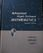Advanced High-School Mathematics (David B. Surowski)

This book is an advanced mathematics textbook accessible by and interesting to a relatively advanced high-school student. Addressing the direction of high school mathematics in the 21st century,

•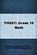FHSST Mathematics for Grade 10 - 12

This book is a very thorough mathematics book for grade 10 - 12 but also has stategies for successful teaching and offers methods to help the student with problem solving and critical thinking skills.

•Understanding Algebra (James W. Brennan)

An brief overview of pre-algebra and introductory algebra topics, written in a style meant to give you the basic facts in a way that is easy to understand, suitable for high-school Algebra I, or for anyone who wants to learn introductory algebra.

•Elementary Algebra (John Redden)

This textbook is the first part, written in a clear and concise manner, making no assumption of prior algebra experience. It carefully guides students from the basics to the more advanced techniques required to be successful in the next course.

•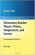Elementary Number Theory: Primes, Congruences, and Secrets

This is a book about prime numbers, congruences, secret messages, and elliptic curves that you can read cover to cover. Many numerical examples are given throughout the book using the Sage mathematical software.

•An Introduction to the Theory of Numbers (Leo Moser)

This book, which presupposes familiarity only with the most elementary concepts of arithmetic (divisibility properties, greatest common divisor, etc.), is an expanded version of a series of lectures for graduate students on elementary number theory.

•Topology of Numbers (Allen Hatcher)

A textbook on elementary number theory from a geometric point of view, as opposed to the usual strictly algebraic approach. A fair amount of the book is devoted to studying Conway's topographs associated to quadratic forms in two variables.

•Trigonometric Delights (Eli Maor)

This book presents both a survey of the main elements of trigonometry and a unique account of its vital contribution to science and social development.

•Elementary Algebra (Denny Burzynski, Wade Ellis)

This is a textbook that covers the traditional topics studied in a modern elementary algebra course. It is intended for students who (1) have no exposure to elementary algebra, (2) have previously had an unpleasant experience with elementary algebra, or (3) need to review algebraic concepts and techniques.

•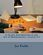A Gentle Introduction to the Art of Mathematics (Joseph Fields)

The purpose of this book is to acclimatize the student to some of the culture and terminology of mathematics and to begin developing in them a proficiency at reading and writing mathematical proofs.

•Precalculus: An Investigation of Functions (David Lippman, et al)

Problem solving and mathematical modeling are introduced early and reinforced throughout, providing students with a solid foundation in the principles of mathematical thinking.

•Master Math: Solving Word Problems (Brita Immergut)

This book is a comprehensive reference guide that explains and clarifies the difficulties people often face with word problems, in a simple, easy-to-follow style and format.

•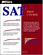Nova's SAT Prep Course (Jeff Kolby)

This book is aimed to test-prep companies to prepare for the new SAT. Many of the exercises in this book are designed to prompt you to think like an SAT test writer. They illustrate the process of creating SAT questions.

•Lecture Notes on Mathematical Olympiad Courses: For Junior

This book is based on the lecture notes of the mathematical Olympiad training courses conducted by the author in Singapore. It introduces a variety concepts and methods in modern mathematics.

•Mathematical Olympiad in China: Problems and Solutions (Xiong)

This book comprises a collection of original problems with solutions that China used to train their Olympiad team in the years from 2003 to 2006.

•How to Help Your Child Excel in Math: An A-Z Survival Guide

The book is an alphabetical dictionary and handbook that gives parents of elementary, middle school, and high school students what they need to know to help their children understand the math they're learning.

Book Categories
 :All CategoriesTop Free BooksRecent BooksMiscellaneous BooksComputer EngineeringComputer LanguagesComputer ScienceData Science/DatabasesJava and Java EE (J2EE)Linux and UnixMathematicsMicrosoft and .NETMobile ComputingNetworking and CommunicationsSoftware EngineeringSpecial TopicsWeb Programming
Other Categories